# NCERT Solutions Class 9 Maths Chapter 4 Linear Equations in Two Variables

Here you can get free NCERT Solutions for Class 9 Maths, of Chapter 4 Linear Equations in Two Variables. All NCERT Book Solutions are given here exercise wise for Linear Equations in Two Variables. NCERT Solutions are helpful in the preparation of several school level, graduate and undergraduate level competitive exams. Practicing questions from NCERT Maths Book, its Solutions for Class 9 Chapter 4 Linear Equations in Two Variables is proven to enhance your subject skills.

 Class: 9th Class Chapter: Chapter 4 Name: Linear Equations in Two Variables Subject: Maths

## NCERT Solutions Class 9 Maths Chapter 4 – Linear Equations in Two Variables

NCERT Class 9 Solutions Chapter 4 – Linear Equations in Two Variables are given below.

NCERT Solutions for Class 9 Maths Chapter 4 Linear Equations in Two Variables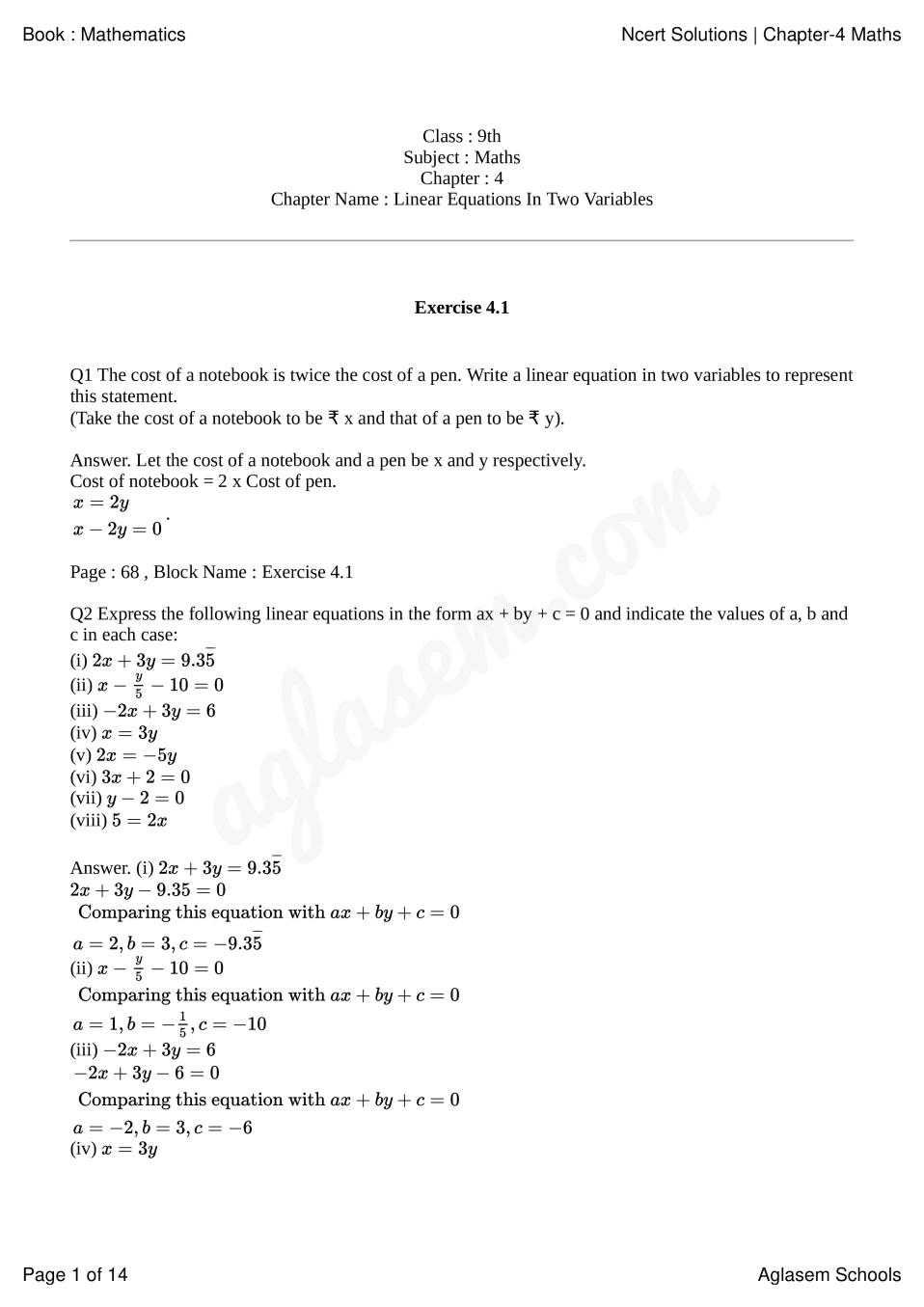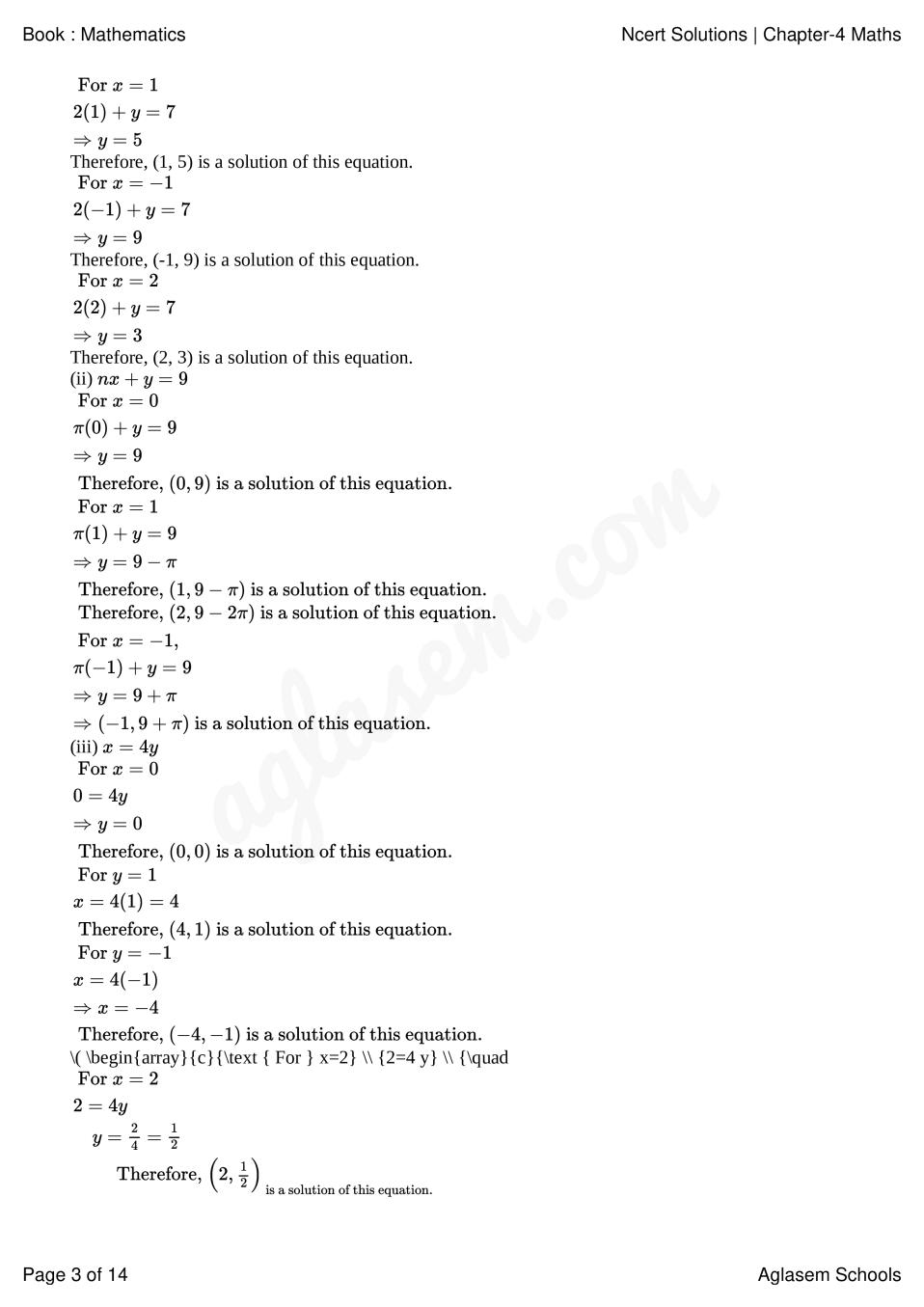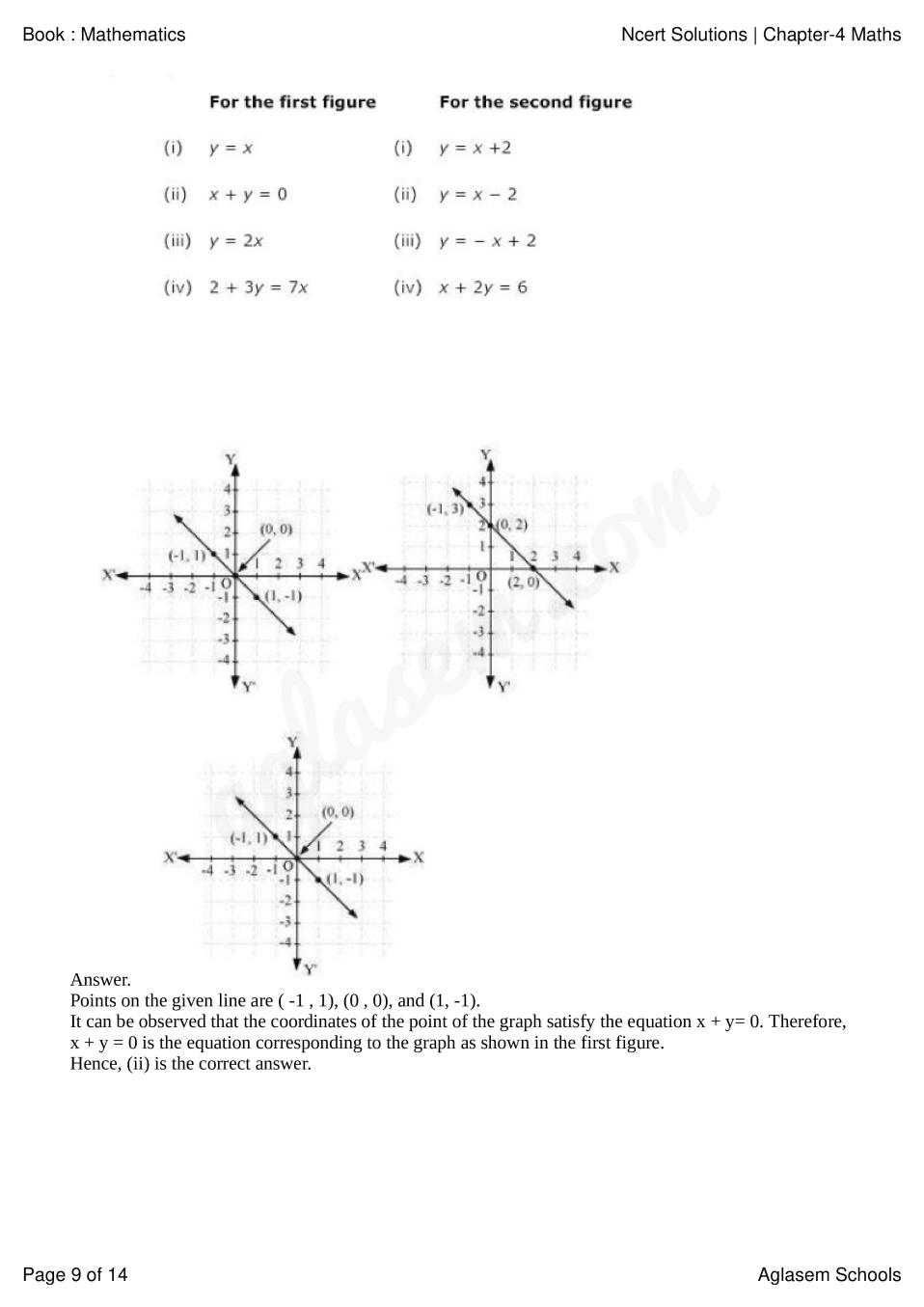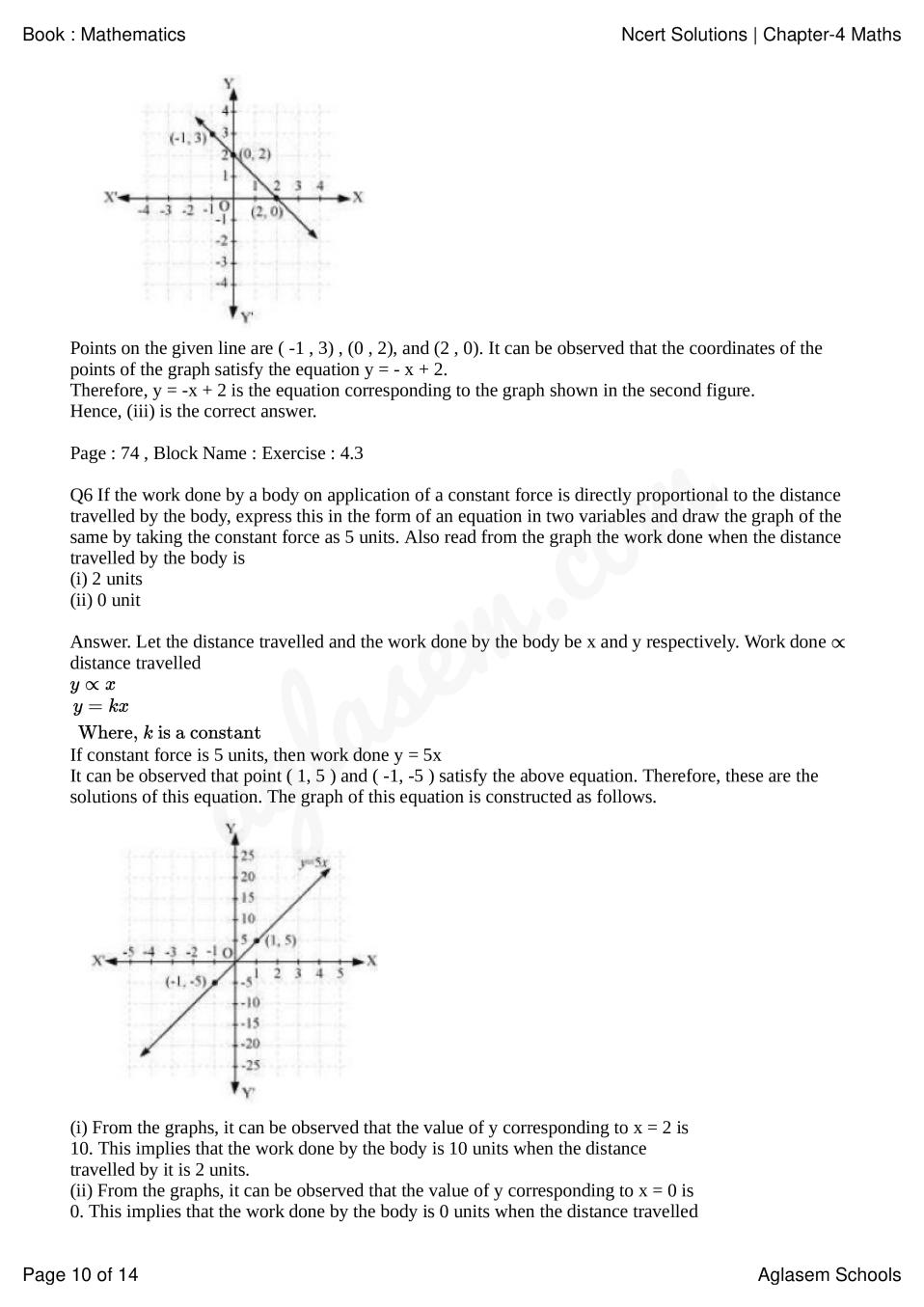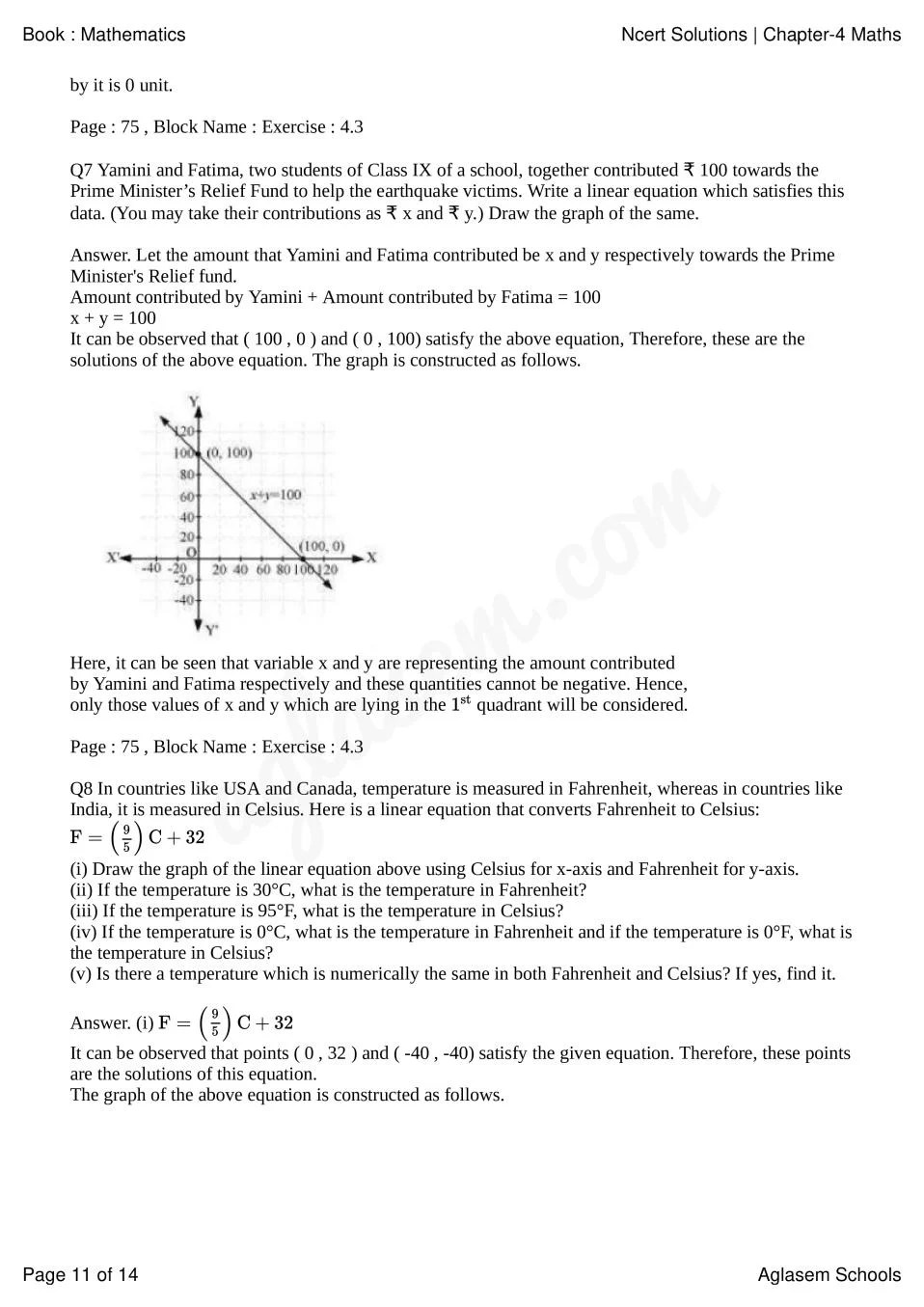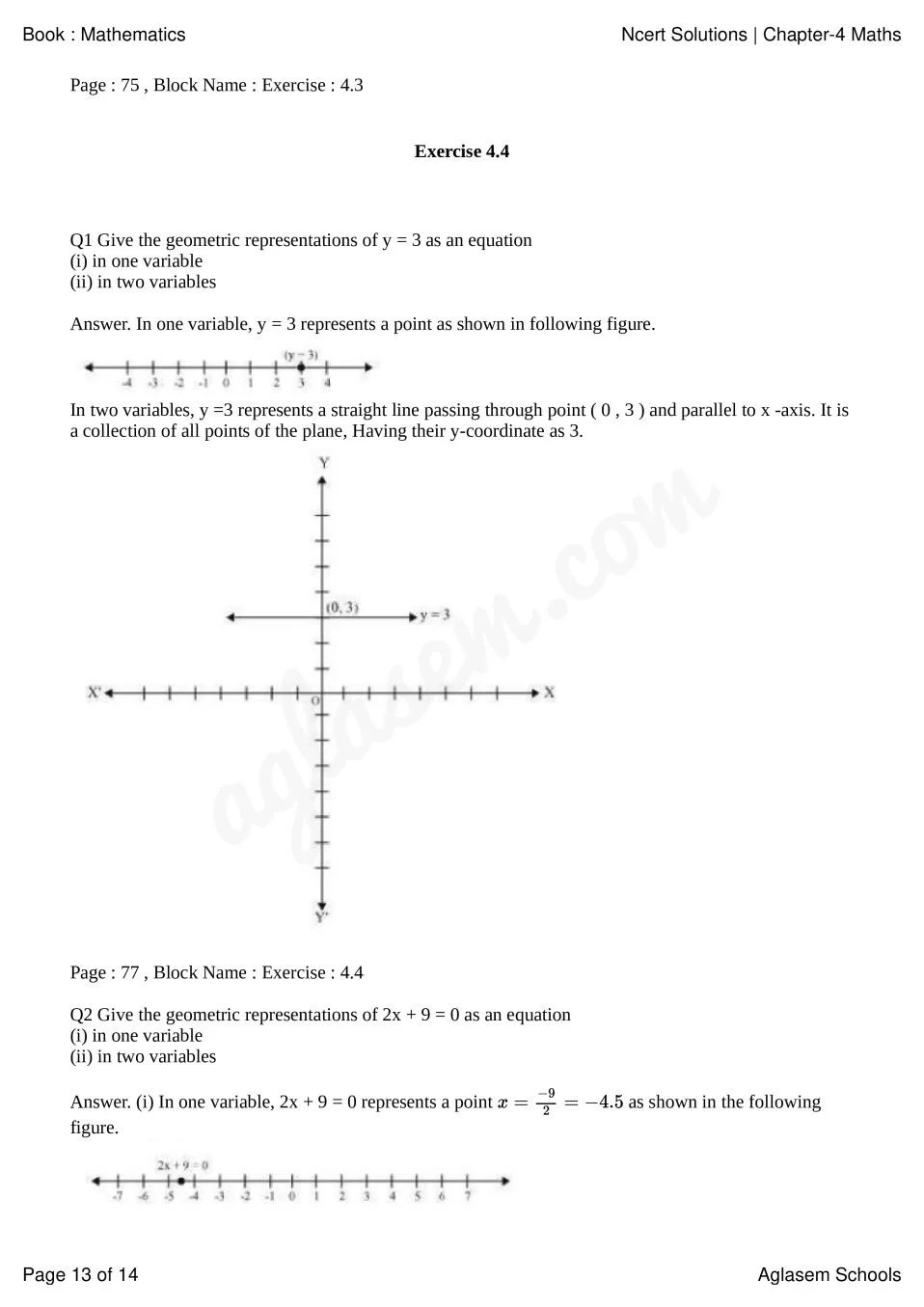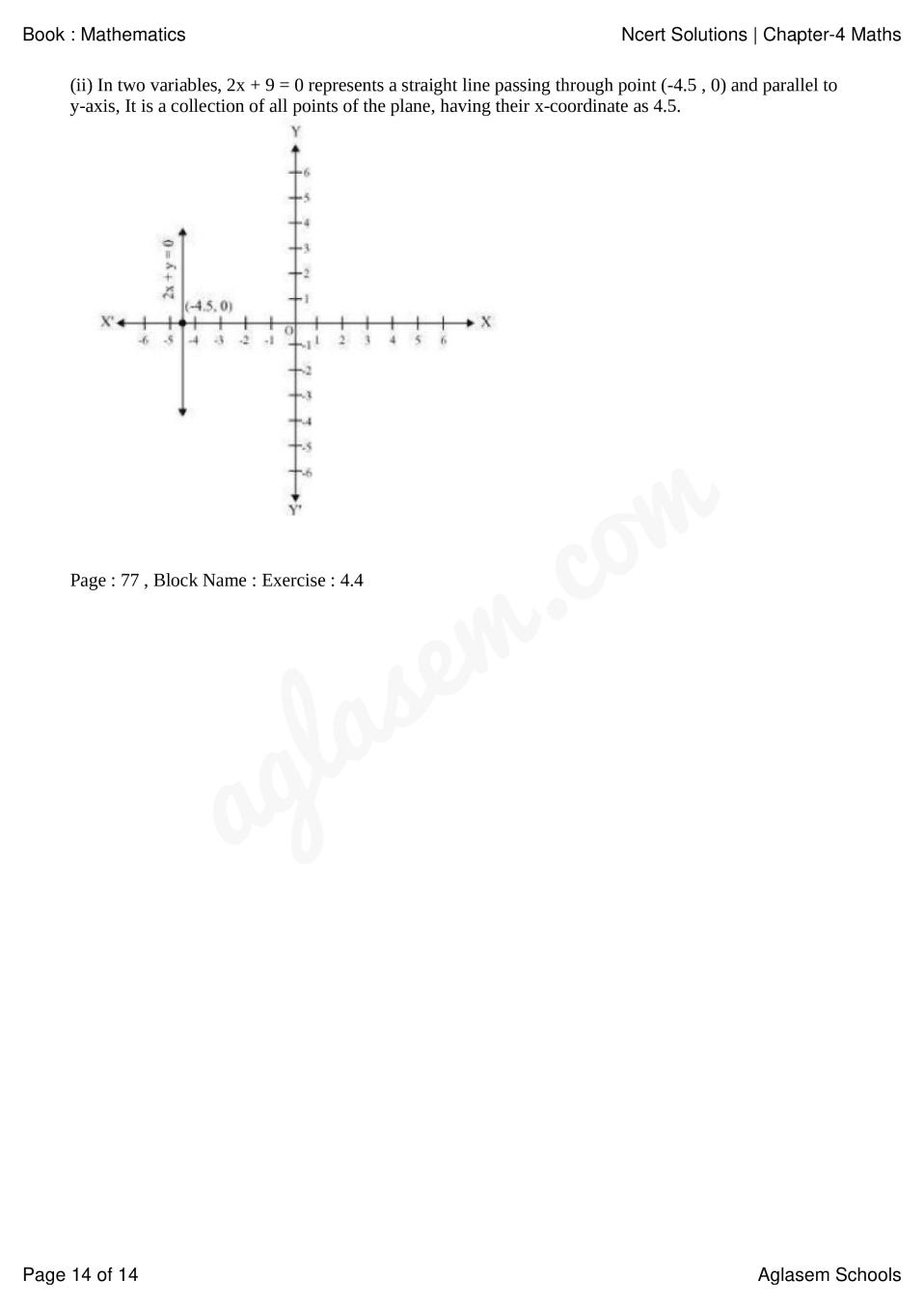### Class 9 Maths Chapter 4 NCERT Solutions

Get step by step solutions for the questions given in class 9 Maths textbook from the latest NCERT as per board exam guidelines.

Exercise Covered under Maths Book Class 9 Maths Chapter 4 NCERT Solutions

Here on this page we have covered following exercises from NCERT Maths Book for Class 9 Maths Chapter 4 Linear Equations in Two Variables

• Exercise: 4.1
• Exercise: 4.2
• Exercise: 4.3
• Exercise: 4.4

We also suggest you to practice all the solved examples given in the NCERT book to clear your concepts on Linear Equations in Two Variables. You can buy NCERT Books for Class 9 Maths online for doorstep delivery.

### NCERT Solutions Class 9 Chapter Wise

If you want NCERT solutions of any topic other than Chapter 4 Linear Equations in Two Variables, check it from here. Maths Book by NCERT has all chapters that are in your Class 9 syllabus.

### NCERT Solutions Class 9 All Subjects

All NCERT Solutions for Subjects other than Maths are provided below:

 English Hindi Science Maths Social Science

### Other Classes NCERT Solutions

If you want NCERT solutions of any class other than Class 9, check it from here.